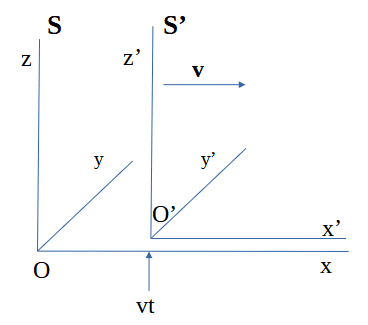# Only Minkowski or Galilei from commutative Velocity Composition

• I
• Sagittarius A-Star

#### Sagittarius A-Star

TL;DR Summary
Derive Lorentz transformation from SR postulate 1 (principle of relativity), assuming linearity and assuming, that velocity composition is commutative (if GT can be excluded)

In the paper "Nothing but Relativity" from Palash B. Pal, they use instead the group law, that two consecutive boosts in the same direction must yield again a boost (equations 24 and 25).
https://arxiv.org/abs/physics/0302045

Why does it follow from the group law, that velocity composition is commutative?
The LT can be derived from the first postulate of SR, assuming linearity an that velocity composition is commutative, and that GT can be excluded: ##t' \neq t##.Definition of the constant velocity ##v##:

##x' = 0 \Rightarrow x-vt=0\ \ \ \ \ \ ##(1)

With assumed linearity follows for the only possible transformation, that meets requirement (1), where ##A_v## may be a function of the constant velocity ##v##:

##\require{color} x' = \color{red}A_v(x-vt)\color{black}\ \ \ \ \ \ ##(2)

With SR postulate 1 (the laws of physics are the same in all inertial reference frames) follows, that the inverse transformation must have the same form, if the sign of ##v## is reversed:

##\require{color}x = A_v(\color{red}x'\color{black}+vt')\ \ \ \ \ \ ##(3)

Eliminating ##x'##, by plugging the right-hand side of equation (2) for ##\require{color} \color{red}x'\color{black}## into (3), and resolving (3) for ##t'## yields the transformation formula for time:

##t' = A_v(t-x\frac{1-\frac{1}{A_v^2}}{v})\ \ \ \ \ \ ##(4)

The velocity composition formula follows by calculating ##dx'/dt'## from equations (2) and (4), with ##u=dx/dt##:

##u' = dx'/dt' = \frac{A_v(dx-vdt)}{A_v(dt-dx\frac{1-1/A_v^2}{v})} = \frac{u-v}{1-u(1-1/A_v^2)/v}\ \ \ \ \ \ ##(5)

With assuming, that velocity composition is commutative, follows from (5):

##u \oplus (-v) = (-v) \oplus u##

##\frac{u-v}{1-u(1-1/A_v^2)/v} = \frac{(-v)+u}{1-v(1-1/A_u^2)/u} ##

## u(1-1/A_v^2)/v = v(1-1/A_u^2)/u \ \ \ \ \ \ ##(6)

Now I sort equation (6) in such a way, that the left side depends only on ##v## and the right side only on ##u##. This can only be, if both sides are constant (overall independent of the velocities).

##(1-1/A_v^2)/{v}^2 = (1-1/A_u^2)/{u}^2 := \alpha##
##\Rightarrow##
##A_v= \frac{1}{\sqrt{1-\alpha v^2}}\ \ \ \ \ \ ##(7)

Plugging the the right-hand side of (7) for ##A_v## into (2), (4) and (5) yields transformation formulas, now containing a yet to be determined constant ##\alpha##, that does not depend on the velocity ##v##:
$$x' = \frac{1}{\sqrt{1-\alpha v^2}} (x-vt)\ \ \ \ \ \ \text{(8)}$$
$$t' = \frac{1}{\sqrt{1-\alpha v^2}}(t-vx \alpha)\ \ \ \ \ \ \text{(9)}$$
$$u' = \frac{u-v}{1-uv \alpha}\ \ \ \ \ \ \text{(10)}$$
These are the only possible transformation formulas, which fulfill SR postulate 1 (the laws of physics are the same in all inertial reference frames), linearity and that velocity composition is commutative. SR postulate 2 (the vacuum speed of light is the same in all inertial frames) was not used.

Only one of the following three cases can be valid:
1. ##\alpha < 0##
2. ##\alpha = 0##
3. ##\alpha > 0##
Case 1 can be excluded because of missing causality-invariance, see the linked paper "Nothing but Relativity".
Case 2, the GT, can be excluded when assuming ##t' \neq t##, which is the opposite of Newton's assumption of an "absolute time" (see equation 9).

Then, only case 3 can be valid. Equation (10) shows, that ##\alpha## must have as unit the inverse of the square of a velocity. Therefore, I can set

##\alpha := 1/c^2\ \ \ \ \ \ ##(11)

Then setting ##u := c## and ##\alpha := 1/c^2## in equation (10) shows, that ##c## is an invariant velocity. Experiments showed, that light moves with that invariant velocity.

Plugging the right-hand side of (11) for ##\alpha## into (8) and (9) yields the LT.

https://arxiv.org/abs/1504.02423

Why follows from the group law, that velocity composition is commutative?

Last edited:
•Dale and vanhees71

•